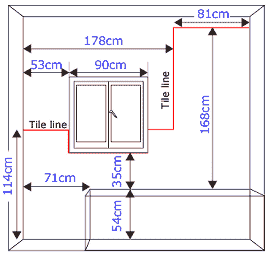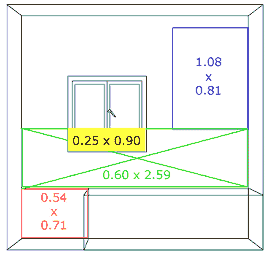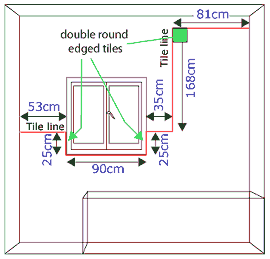# Estimating ceramic tiles for walls.

Estimating the number of tiles required for a job is important to ensure that you have just enough tiles; not too few and not too many. If your supplier will take back unused tiles this makes life easier but you still don't want to have too few to start with - if you have to go back for some more of a pattern, the chances are that those you buy will be of a different batch and so the colours may vary slightly from the previous ones.

This page deals with calculating the number of wall tiles required to tile a room, it includes a worked example and a table giving wall coverage for different size tiles (both in metric and imperial). It includes consideration of frieze tiles and individual decorative tiles.

While this page uses an example with metric measurements, the principle is the same when working in imperial.

Begin to work out the area of tiling required by deciding on the area of each wall you want to tile.Around a shower, plan to tile to at least 15cm (6 inches) above the shower-head behind it and on any adjacent walls at least 75cm (30 inches) from the corner of the shower head wall.

See our page on setting out tiling for some suggestions on how to achieve a tile line which will make the actual tiling easier.

Measure the height and width of the tile area to the various edges - wall corners, fittings, windows etc. .Divide up the area into rectangular areas

If you intend to use narrow frieze tiles around the room, reduce the height of each rectangle containing the frieze by the intend height of the frieze tiles - i.e. if the frieze will run just under the window in the illustration, just reduce the green area height. If using full size frieze tiles, do not make any adjustment to the figures.

For each area, multiply the height by the width.

With irregular sections on one wall, either break it down into smaller rectangular shapes or measure a larger rectangle and take away the unwanted area from it - the latter technique is used in the example by subtracting the area of the window which projects into the green area (i.e. the yellow area is subtracted from the green area).

For this example:

Red area: 0.54 x 0.71 = 0.38 sq m

Green area: 0.60 x 2.59 = 1.55 sq m

less 0.25 x 0.90 (the window) = 1.33 sq m

Blue area: 1.08 x 0.81 = 0.88 sq m

Now work out the area for any tiled surfaces other than the wall face - in this example, the bottom and sides of the window recess which will be tiled up to the tile line:

(0.25 + 0.90 + 0.25) * 0.075 = 0.11 sq m

(that is, the length of the window edge to be tiled multiplied by the depth of the recess)

Adding all these areas together gives a grand total of :

0.38 + 1.33 + 0.88 + 0.11 = 2.7 sq metres

That is one wall done. Now repeat it for any other walls and add the totals together. Lets say, that the total tiled area for all the walls in the room is 8.5 sq metres.

The number of tiles you will need depends, obviously, upon the size of tiles you wish to use. This table gives approximate numbers of tile coverage for different tile sizes:

 Tile size (mm x mm) Number of tiles per square metre Tile size (inch x inch) Number of tiles per square yards 100 x 100 100 4 x 4 84 150 x 75 88 6 x 3 74 150 x 150 44 6 x 6 37 200 x 100 50 8 x 4 41 200 x 200 25 8 x 8 21 200 x 250 20 8 x 10 17 200 x 300 17 8 x 12 14 250 x 330 12 10 x 13 10

Assuming that you are going to use 150 x 150 mm tiles, to cover the 8.5 sq metres you have calculated, you will need (8.5 x 44) 374 tiles.

BUT, you if you want to use edging, full size frieze or individual decorative tiles, this number may be reduced.

## Edging tilesIf using edging tiles (rather than edging strip), the number of tiles required needs to be worked out.

Measure the length of the tile edges for the whole room (one wall shown right).

Identify the number of double round edged tiles required, in this illustration that will be 3 - one for each side of the window recess, and one where the wall tiling turns down. Reduce each dimension affected by the position of a double round edge tile by the width of a tile.

For each of the dimensions measured, divide it by the width of the tiles to find the number of single round edge tiles required - round up the result to a complete tile.

 edge run for single round edge tiles number of 150mm tiles rounded up 530 3.5 4 100 (250 - 150) 0.67 1 900 6 6 100 (250 - 150) 0.67 1 350 2.3 3 1530 (1680 - 150) 10.2 11 660 (810 - 150) 4.4 5 Total 31

Having worked out the edging tiles for all walls, let say you arrive at:

• 8 double round edged tiles
and
• 96 single round edged tiles

## Frieze tiles

Whether you are using narrow frieze tiles, or full size frieze tiles, you will need to calculate how many you require. Simply measure the length of the tile run for these tiles, and divide it by the width of the tiles being used. Round up the figure and do each wall separately. Then add the figures for each wall together.

In the above example, with the frieze tiles below the window, the tile run is 2.59 metres and using 150mm wide tiles this equates to 18 frieze tiles.

Lets say, that for all walls a total of 50 frieze tiles are required.

## Individual decorative tiles

Similar to frieze tiles, the number of individual decorative tiles need to be worked out. This is not so easy as you have to imagine how they will look and where they will go, it is easier where a whole wall is tiled as you could decide "every 5th tile on every third row".

With partial wall tiling, it does help to draw out the individual tiles on each wall and mark those to be individual decorative tiles, not only does this help find how many you require, but it can also help when you are doing the actual tiling.

Lets say, that for all walls a total of 20 individual decorative tiles are required.

## The final calculation of tile numbers

By taking the numbers of any round edged tiles (both double and single round edged), frieze (if full size) and individual decorative tiles away from the original calculation for the whole tiling area, this gives a requirement of:

• 200 'plain' field tiles (if full frieze tiles are being used, otherwise 250).
• 8 double round edged tiles
• 96 single round edged tiles
• 50 frieze tiles.
• 20 individual decorative tiles.

Remember: only deduct the number of frieze tiles if full frieze tiles are being used, otherwise the number of frieze tiles calculated is the number of the narrow type required.

To these figures we need to add five percent for wastage (rounding up the calculations). If you are not very experienced with cutting tiles, it may be worth adding ten percent instead of five. Wastage will result from having to cut tiles to size, while some off cuts may be usable elsewhere on the walls, some will not. In addition, a few tiles will generally be broken whilst cutting.

So the final totals required for each tile type are (using 5% for wastage) is:

• 210 'plain' field tiles.
• 9 double round edged tiles
• 101 single round edged tiles
• 53 frieze tiles.
• 21 individual decorative tiles - just one spare may not be enough, so take care when cutting them, place them where you will not need to cut them, or increase the number to 22.